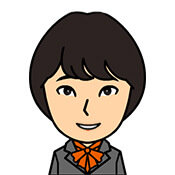When it comes to a little math and numbers, it is useful to be able to speak that in Korean.

Let’s practice reading simple mathematical formulas in Korean.

### Addition is “덧셈”, sum is “합”.

Addition is speaked 덧셈 in Korean.덧셈은 수학의 기본이 되는 연산입니다.
Addition is a fundamental mathematical operation.

There are also words such as 합산(合算) and 가산(加算), but the most commonly used is 덧셈.200과 35의 은 235예요.
The sum of 200 and 35 is 235.

And there is also a word which means the sum of addition.

We use 더하다 to express adding a number.100에 300을 더하면 400입니다.
Add 300 to 100 and you get 400.775랑 8을 더하면 783이 됩니다.
If you add 775 and 8, you get 783.

It does not use difficult grammar and can be expressed as long as you know how to read numbers.

The addition sign “+” is speaked 더하기.98 + 62 = 160
구십팔 더하기 육십이 백육십35 + 21 = 56
삼십오 더하기 이십일 오십륙

더하기 is the noun form of 더하다 with “-기”.

Also, equal “=” is speaked -은/는.

## The subtraction.

### Subtraction is “뺄셈”, difference is “차”.

Subtraction is speaked 뺄셈 in Korean.뺄셈도 덧셈만큼 중요합니다.
Subtraction is just as important as addition.

There are also words like 감산(減算), but let’s learn 뺄셈 first.75와 32의 는 42예요.
The difference between 75 and 32 is 42.

The word for the subtraction answer is .

### Subtracting number is “빼다”.

We use 빼다 to express subtract a number.50에서 2를 빼면 48이 됩니다.
Subtract 2 from 50 and you get 48.1000에서 250을 빼면 나머지는 750이에요.
If you take 250 out of 1000, the remainder is 750.

The way it is expressed will be similar to addition.

### How to read subtraction symbols.

The subtraction sign “-” is speaked 빼기.1234 34 = 1200
천이백삼십사 빼기 삼십사는 천이백313  76 = 237
삼백십삼 빼기 칠십육은 이백삼십칠

빼기 is the noun form of 빼다 by -기.

민수네 집에서 지하철역까지 100m입니다. 민수는 집에서 지하철역을 향해 35m를 걸었습니다. 지하철역까지 남은 거리가 얼마인가?

답：

Since this is an exercise in reading formulas, you do not have to be able to do the problems.

## The multiplication.

### Multiplication is “곱셈”, product is “곱”.

Multiplication is speaked 곱셈 in Korean.곱셈은 초등학교 2학년 때 공부했어요.
I studied multiplication in second grader.

There is a word 승산(乗算) in multiplication, but for now, let’s learn 곱셈.24와 3의 은 72예요.
The product of 24 and 3 is 72.

The word for the multiplication answer is 곱.

### Multiplicating number is “곱하다”.

We use 곱하다  to express multiplicat a number.280에 3을 곱하면 840이에요.
Multiply 280 by 3 and you get 840.72에 0.1을 곱하면 7.2가 됩니다.
Multiply 72 by 0.1 to get 7.2.

Since the method of expression is the same as that of addition and subtraction, we will omit the details.

### How to read multiplication symbols.

The multiplication sign “×” is speaked 곱하기.75 × 2.4 = 180
칠십오 곱하기 이점사는 백팔십25 × 2 = 45
오분의 이 곱하기 이는 오분의 사

You can use 곱하다 as 곱하기 with -기.

Then, let’s try to solve a math problem in Korean.

a=3, b=1012일 때 다음의 값을 구하시오.

a×b=?

답：

## The division.

### Division is “나눗셈” and quotient is “몫”.

Division is speaked 나눗셈 in Korean.곱셈을 못 하면 나눗셈도 못해요.
If you can’t multiply, you can’t divide.

There is also a kanji word 제산 (除算), but it is usually fine to use 나눗셈.24와 3의 은 8이에요.
The quotient of 24 and 3 is 8.

The word for the division answer is .

몫 also means “share or portion”, and a piece of pizza or cake cut into pieces by several people is also “몫”.

### Divisioning number is “나누다”.

We use 나누다  to express division a number.21을 3으로 나누면 7이 나옵니다.
Dividing 21 by 3 yields 7.58를 14로 나누면 4와 나머지 2가 됩니다.
Dividing 58 by 14 yields 4 and not much 2.

The way division is expressed is the same as in other calculations.

### How to read division symbols.

The division sign “÷” is speaked 나누기.100 ÷ 25 = 4
나누기 이십오는 사23 ÷ 2 = 13
삼분의 이 나누기 이는 삼분의 일

The noun form of 나누다 is 나누기.

There is no need to solve difficult calculation problems, just practice reading.